• 2021-05-23 06:32:05

共回答了19个问题采纳率：89.5%

牛顿迭代法

牛顿迭代法又称牛顿切线法,它采用以下方法求根：先任意设定一个与真实的根接近的值x0作为第一个近似根,由x0求出f(x0),过(x0,f(x0))点做f(x)的切线,交x轴于x1,把它作为第二次近似根,再由x1求出f(x1),再过(x1,f(x1))点做f(x)的切线,交x轴于x2,再求出f(x2),再作切线……如此继续下去,直到足够接近真正的x为止.

其中f'(X0)是函数在X0处的斜率,也就是在X0处的导数.

代码如下：

#include

#include

float f(float a,float b,float c,float d,float x)

{

float f;

f=((a*x+b)*x+c)*x+d;

return f;

}

float f1(float a,float b,float c,float x)

{

float f;

f=(x*3*a+2*b)*x+c;

return f;

}

float root(float a,float b,float c,float d)

{

float x0,x1=1;

do

{

x0=x1;

x1=x0-f(a,b,c,d,x0)/f1(a,b,c,x0);

}while(fabs(x1-x0)>=1e-6);

return x0;

}

void main()

{

float a,b,c,d,x;

printf("input four float numbers:n");

scanf("%f%f%f%f",&a,&b,&c,&d);

x=root(a,b,c,d);

printf("%.1fX^3+%.1fX^2+%.1fX+%.1f=0 its root near x=1.5 is :%.4fn",a,b,c,d,x);

getch();

}

1年前

9

更多相关内容
• 牛顿迭代法定义如下（来自百度百科）： 用牛顿迭代法小试牛刀用来求解一元次方程的根（工程下载地址【注：不好意思，下载题目写成了二元一次方程，见谅。】，release下的应用程序可以直接运行），代码如下：...

牛顿迭代法定义如下（来自百度百科）：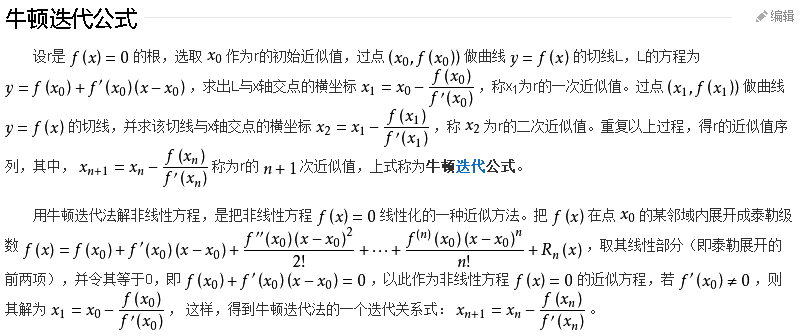用牛顿迭代法小试牛刀用来求解一元二次方程的根（工程下载地址【注：不好意思，下载题目写成了二元一次方程，见谅。】，release下的应用程序可以直接运行），代码如下：

typedef struct _MyP
{
float x;
float y;
}MyP;

// C++实现牛顿迭代法求一元二次方程的解
// 设一元二次方程的通用方程为 y = ax^2 + bx + c
// 则其导函数为 y = 2ax + b

// 1、构造方程
float accuracy = 0.001;
float a = -5;
float b = 4;
float c = 0;

// 2、找极值点，令导函数 y = 2ax + b = 0，求的极值点坐标P.x = -b/2a, P.y = aP.x^2 + bP.x + c
MyP P;
MyP PTemp;
P.x = (-1 * b) / (2 * a);
P.y = (a * P.x * P.x) + (b * P.x) + c;

// 3、判断定义域在(-无穷大, P.x)和在定义域(P.x, +无穷大)的单调性和开口方向
PTemp.x = P.x - 10;
PTemp.y = (a * PTemp.x * PTemp.x) + (b * PTemp.x) + c;
if (PTemp.y > P.y){ // 在(-无穷大, P.x)单调递减，在(P.x, +无穷大)单调递增，开口向上

ui.tbResult->append(QString::fromLocal8Bit("开口方向：向上"));
// 4.1、判断方程是否有解，有解的话几个解
if (P.y > 0){

ui.tbResult->append(QString::fromLocal8Bit("解集情况：无解"));
}
else if (P.y == 0){

ui.tbResult->append(QString::fromLocal8Bit("解集情况：1个解"));
ui.tbResult->append(QString::fromLocal8Bit("解1：") + QString("(%1, %2)").arg(P.x).arg(P.y));
}
else{

ui.tbResult->append(QString::fromLocal8Bit("解集情况：2个解"));
// 5.1、牛顿迭代求解
float k;		// 切点斜率
MyP P1, P2;
P1.x = P.x + accuracy;	// 设置迭代起始点1
P2.x = P.x - accuracy;	// 设置迭代起始点2
float x1, x2;	// 解1和解2

while(1){

k = (2 * a * P1.x) + (b);					// 求切点斜率
x1 = P1.x - (P1.y / k);						// 切线与X轴的交点的x坐标
P1.y = (a * x1 * x1) + (b * x1) + c;		// 求x1在一元二次方程曲线上的Y值
if (fabs(P1.y) <= 0.001){

if (x1 < accuracy) x1 = 0;
if (P1.y < accuracy) P1.y = 0;
ui.tbResult->append(QString::fromLocal8Bit("解1：") + QString("(%1, %2)").arg(x1).arg(P1.y));
break;
}
P1.x = x1;									// 更新迭代点
}
while(1){

k = (2 * a * P2.x) + (b);					// 求切点斜率
x2 = P2.x - (P2.y / k);						// 切线与X轴的交点的x坐标
P2.y = (a * x2 * x2) + (b * x2) + c;		// 求x1在一元二次方程曲线上的Y值
if (fabs(P2.y) <= 0.001){

if (x2 < accuracy) x2 = 0;
if (P2.y < accuracy) P2.y = 0;
ui.tbResult->append(QString::fromLocal8Bit("解2：") + QString("(%1, %2)").arg(x2).arg(P2.y));
break;
}
P2.x = x2;									// 更新迭代点
}
}
}
else{				// 在(-无穷大, P.x)单调递增，在(P.x, +无穷大)单调递减，开口向下

ui.tbResult->append(QString::fromLocal8Bit("开口方向：开口向下"));
// 4.2、判断方程是否有解，有解的话几个解
if (P.y < 0){

ui.tbResult->append(QString::fromLocal8Bit("解集情况：无解"));
}
else if (P.y == 0){

ui.tbResult->append(QString::fromLocal8Bit("解集情况：1个解"));
ui.tbResult->append(QString::fromLocal8Bit("解1：") + QString("(%1, %2)").arg(P.x).arg(P.y));
}
else{

ui.tbResult->append(QString::fromLocal8Bit("解集情况：2个解"));
// 5.2、牛顿迭代求解
float k;		// 切点斜率
MyP P1, P2;
P1.x = P.x + accuracy;	// 设置迭代起始点1
P2.x = P.x - accuracy;	// 设置迭代起始点2
float x1, x2;	// 解1和解2

while(1){

k = (2 * a * P1.x) + (b);					// 求切点斜率
x1 = P1.x - (P1.y / k);						// 切线与X轴的交点的x坐标
P1.y = (a * x1 * x1) + (b * x1) + c;		// 求x1在一元二次方程曲线上的Y值
if (fabs(P1.y) <= 0.001){

if (x1 < accuracy) x1 = 0;
if (P1.y < accuracy) P1.y = 0;
ui.tbResult->append(QString::fromLocal8Bit("解1：") + QString("(%1, %2)").arg(x1).arg(P1.y));
break;
}
P1.x = x1;									// 更新迭代点
}
while(1){

k = (2 * a * P2.x) + (b);					// 求切点斜率
x2 = P2.x - (P2.y / k);						// 切线与X轴的交点的x坐标
P2.y = (a * x2 * x2) + (b * x2) + c;		// 求x1在一元二次方程曲线上的Y值
if (fabs(P2.y) <= 0.001){

if (x2 < accuracy) x2 = 0;
if (P2.y < accuracy) P2.y = 0;
ui.tbResult->append(QString::fromLocal8Bit("解2：") + QString("(%1, %2)").arg(x2).arg(P2.y));
break;
}
P2.x = x2;									// 更新迭代点
}

}
}

开发环境为VS2013+QT580+OPENCV300，软件运行截图如下：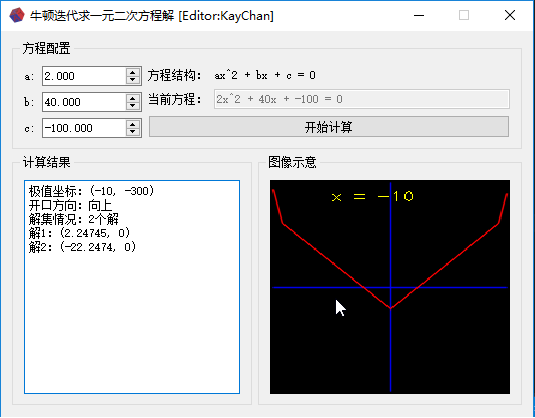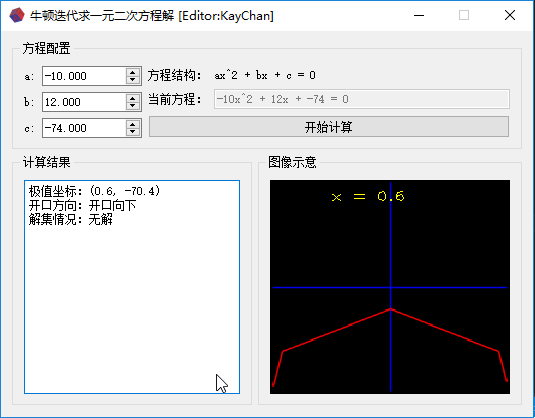工程中的release下的应用程序可以直接运行，需要软件和代码工程的请戳这里

展开全文• 一元线性回归 （1）如何理解“回归分析”？ 回归分析是确定两种或两种以上变量间相互依赖的定量关系的种统计分析方法，是对具有因果关系的影响因素（自变量）和预测对象（因变量）所进行的数理统计分析处理。...

# 学习目标：

## 一.一元线性回归

（1）如何理解“回归分析”？

回归分析是确定两种或两种以上变量间相互依赖的定量关系的一种统计分析方法是对具有因果关系的影响因素（自变量）和预测对象（因变量）所进行的数理统计分析处理。只有当变量与因变量确实存在某种关系时，建立的回归方程才有意义。因此，作为自变量的因素与作为因变量的预测对象是否有关，相关程度如何，以及判断这种相关程度的把握性多大，就成为进行回归分析必须要解决的问题。进行相关分析，一般要求出相关关系，以相关系数的大小来判断自变量和因变量的相关的程度。

（2）分类

标准1：据自变量和因变量之间的关系类型

线性回归分析和非线性回归分析

标准2：按照自变量的数量

一元回归分析和多元回归分析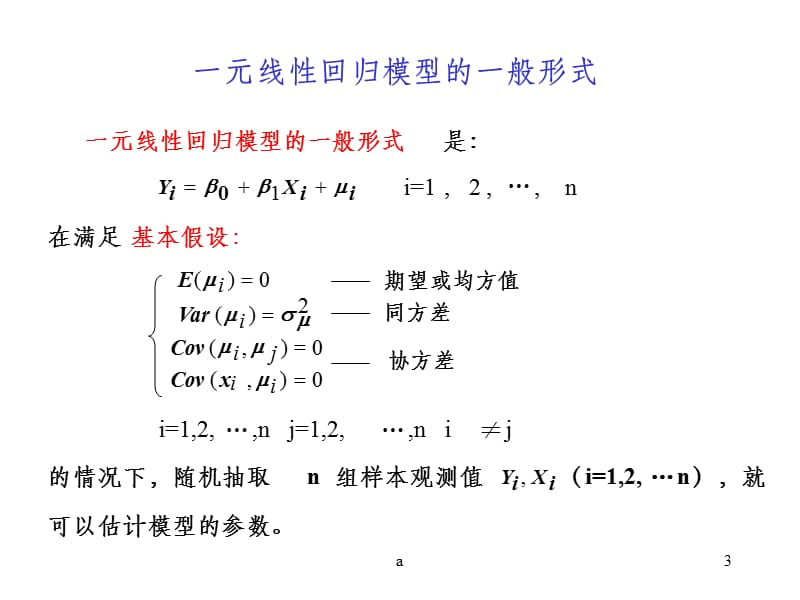一元线性回归的形式为        y = a + b x

根据样本观察数据估计出a和b的数值之后，样本回归方程可作为预测模型，即一元线性回归预    测模型

（3）求解回归预测模型参数的方法

方法一：根据相关系数与标准差求解

直线可以用公式表示：y=bx+a。

回归线斜率m的公式为：b = r *  (SD of y / SD of x)。

转换：x和y值之间的相关系数（r），乘以y值的标准差（SD of y）除以x值的标准偏差（SD of x）。

将   样本的均值点    代入回归线求出  a

相关系数求解公式：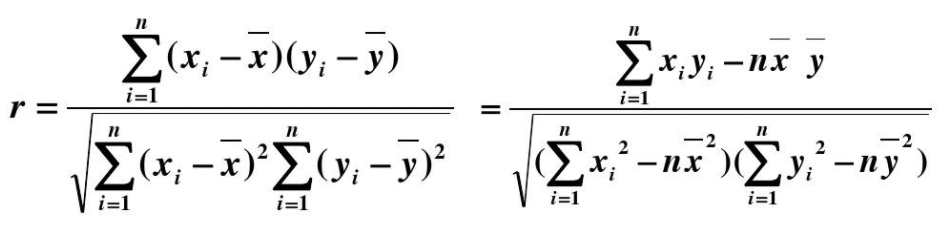## II.梯度下降法

梯度下降原理：

从一条随机线开始，比如说直线a，我们计算这条线的误差平方和，然后调整斜率和y轴截距，重新计算新行的误差平方和。继续调整，直到达到局部最小值，其中平方误差之和最小。

梯度下降法是一种通过多次迭代最小化误差平方和来逼近最小平方回归线的算法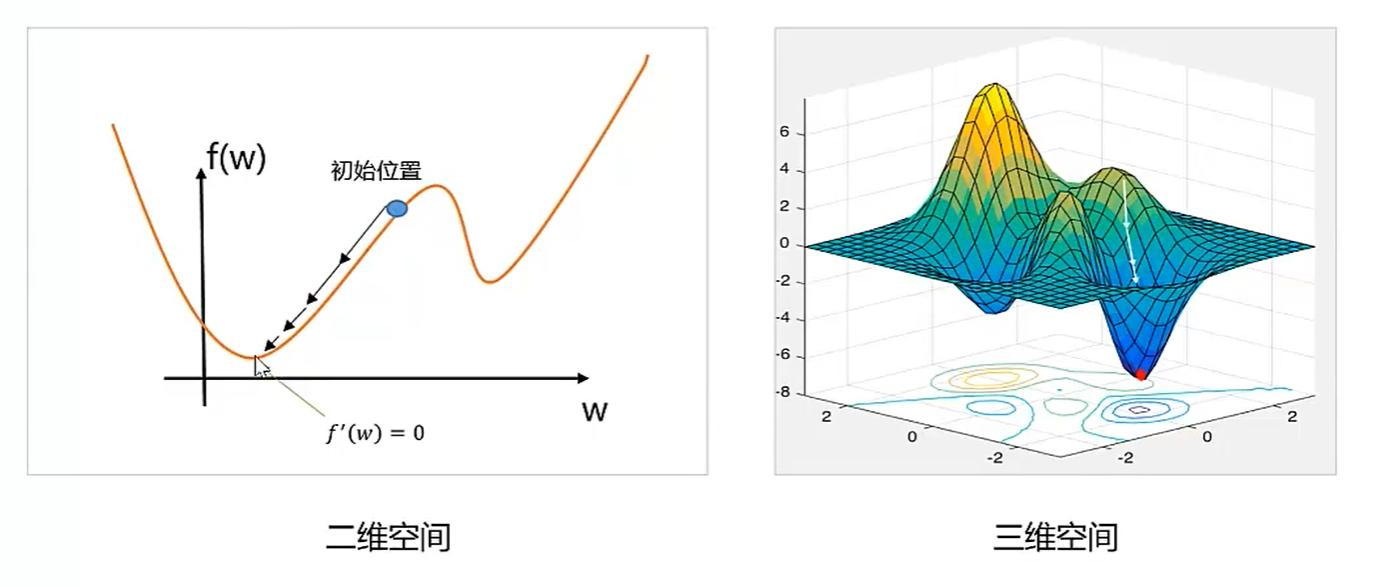成本：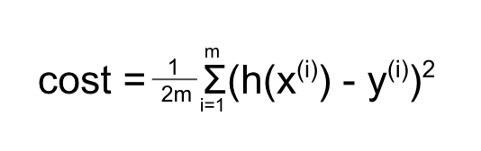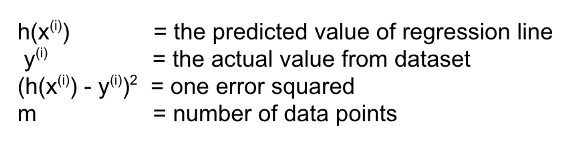“成本”就是误差（预测值-实际值）的平方和

为了是预测模型更加准确（即成本最低），我们可以通过改变斜率和截距来寻找最佳拟合线

如何改变参数呢？

对其求偏导   ，  可以得到下降最快的方向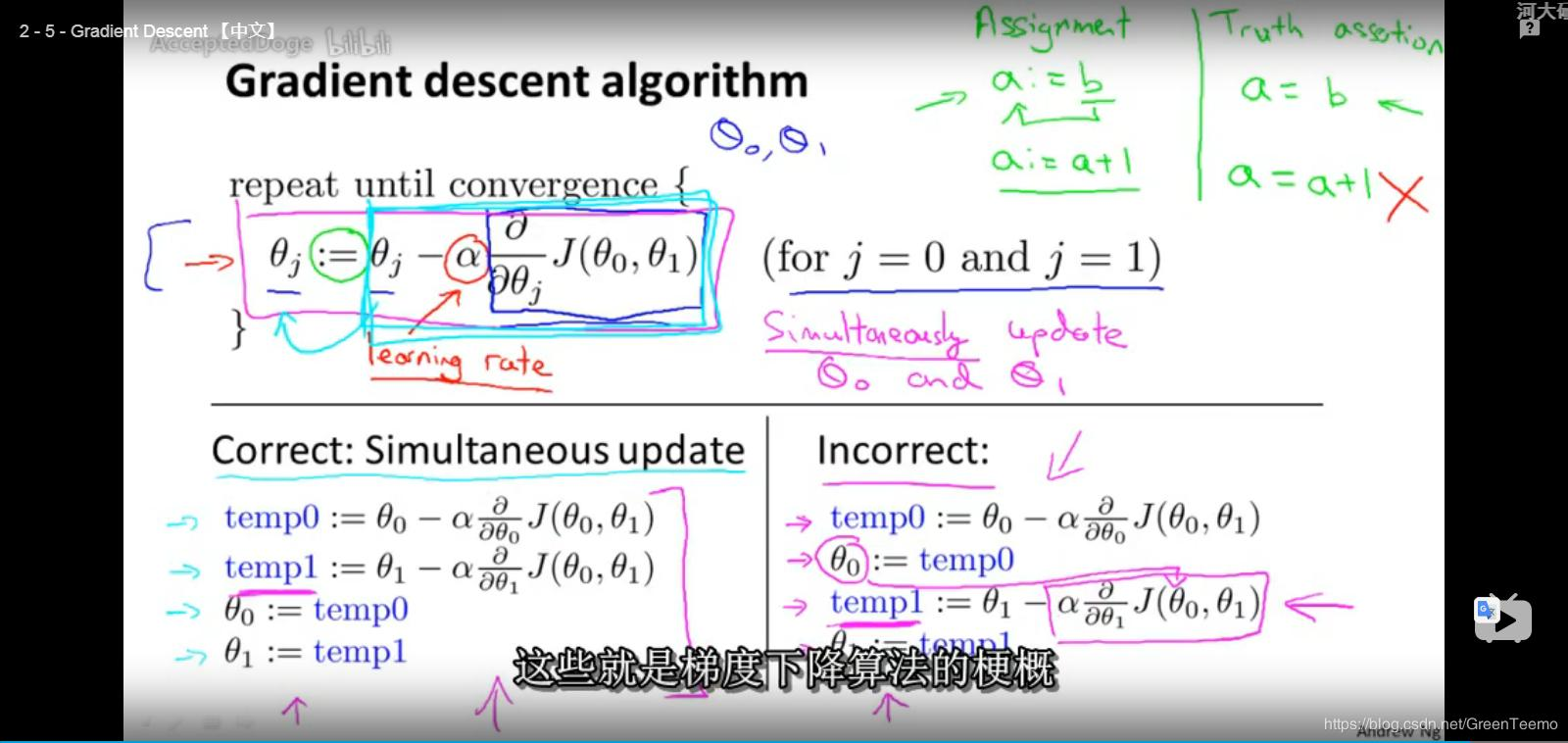我们便引入了梯度下降公式来改变参数值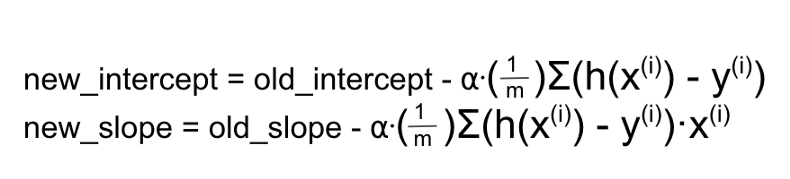关键是选择一个合适的学习速率（α），如果学习速率过小，则会导致收敛速度很慢；如果学习速率过大，那么就会阻碍收敛，即在极值点附近会震荡。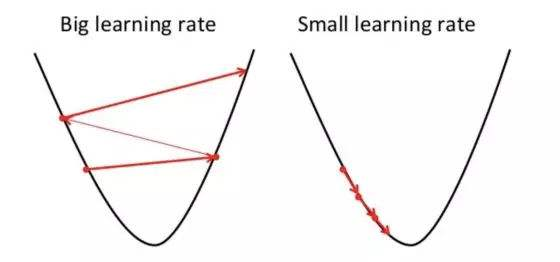学习速率调整（又称学习速率调度，Learning rate schedules），在每次更新过程中，改变学习速率，如退火。一般使用某种事先设定的策略或者在每次迭代中衰减一个较小的阈值。无论哪种调整方法，都需要事先进行固定设置，这便无法自适应每次学习的数据集特点。

（4）求解步骤

• 1、散点图判断变量关系（简单线性）；
• 2、求相关系数及线性验证；
• 3、求回归系数，建立回归方程；
• 4、回归方程检验；
• 5、参数的区间估计；
• 6、预测；

实例如下：

import numpy as np
import matplotlib.pyplot as plt

class SimpleRegress(object):
def __init__(self, x_data, y_data):

self.x_data = x_data
self.y_data = y_data
self.b0 = 0
self.b1 = 1

return

def calculate_work(self):       # 回归方程中b0、b1的求解

x_mean = np.mean(self.x_data)   # x_mean= 14.0
y_mean = np.mean(self.y_data)   # y_mean= 130.0
x1 = self.x_data - x_mean   # x1= [-12.  -8.  -6.  -6.  -2.   2.   6.   6.   8.  12.]
y1 = self.y_data - y_mean   # y1= [-72. -25. -42. -12. -13.   7.  27.  39.  19.  72.]
s = x1 * y1     # s= [864. 200. 252.  72.  26.  14. 162. 234. 152. 864.]
u = x1 * x1     # u= [144.  64.  36.  36.   4.   4.  36.  36.  64. 144.]
self.b1 = np.sum(s) / np.sum(u)      # b1= 5.0
self.b0 = y_mean - self.b1 * x_mean       # b0= 60.0

return

def test_data_work(self, text_data):    # 回归方程的建立与数值预测

result = list([])
for one_test in text_data:
y = self.b0 + self.b1 * one_test
result.append(y)
return result

def root_data_view(self):    # 绘制源数据可视化图
plt.scatter(x_data, y_data, label='simple regress', color='k', s=5)  # s 点的大小
plt.xlabel('x')
plt.ylabel('y')
plt.legend()
plt.show()
return

def test_data_view(self):    # 绘制回归线
# 绘制回归线两个点的数据
x_min = np.min(self.x_data)
x_max = np.max(self.x_data)
y_min = np.min(self.y_data)
y_max = np.max(self.y_data)
x_plot = list([x_min, x_max])
y_plot = list([y_min, y_max])
# 绘制
plt.scatter(x_data, y_data, label='root data', color='k', s=5)  # s 点的大小
plt.plot(x_plot, y_plot, label='regression line')
plt.xlabel('x')
plt.ylabel('y')
plt.legend()
plt.title('simple linear regression')
plt.show()
return

x_data = list([2, 6, 8, 8, 12, 16, 20, 20, 22, 26])
y_data = list([58, 105, 88, 118, 117, 137, 157, 169, 149, 202])
test_data = list()

sr = SimpleRegress(x_data, y_data)
sr.calculate_work()
result = sr.test_data_work(test_data)       # result= [140.0]
#sr.root_data_view()
sr.test_data_view()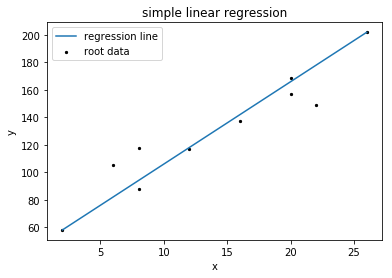展开全文线性回归 回归 算法
• ## 一元三次方程求解

千次阅读 2019-10-18 11:16:05
牛顿迭代法就是不停的用个点的切线拟合曲线，那个点的导数就是切线斜率 依次类推，可以得到求高函数零点的种迭代法： 求n函数零点，需要极值点来划分区间，也就需要求其导数(n-1函数)的零点，依次迭代到n...

a*x^3+b*x^2+c*x+d=0

# 枚举+二分

#include<cstdio>
#include<cmath>
#include<cstdlib>
using namespace std;
const double PI = acos(-1.0);
double a,b,c,d;
double R,m;
double f(double x)
{
return a*x*x*x+b*x*x+c*x+d;
}
//解一元三次方程组 a*x^3+b*x^2+c*x+d=0 ,解的上下界为min_ans,max_ans
double solve(double a,double b,double c,double d,double min_ans,double max_ans)
{
double x,x1,x2,xx;
for (x=min_ans; x<=max_ans; x+=1)
{
x1=x;
x2=x+1;
if(f(x1)==0)//有解
{
if(x1>=0)//判断是否为正数
{
//x1为解
printf("%.2f ",2*R-x1);
return 0;
}
}
else if (f(x1)*f(x2)<0)
{
double l=x1,r=x2;
while (r-l>=0.0001)
{
double mid=(r+l)/2;
if ((f(l)*f(mid))<=0)
r=mid;
else
l=mid;
}
if(l>=0)
{
//l为解
printf("%.2f ",2*R-l);
return 0;
}
}
}
}
int main()
{

scanf("%lf%lf",&R,&m);
a=PI;
b=-3*PI*R;
c=0;
d=(3)*m;
solve(a,b,c,d,-100,100);
return 0;

}


# 盛金公式(求三个根）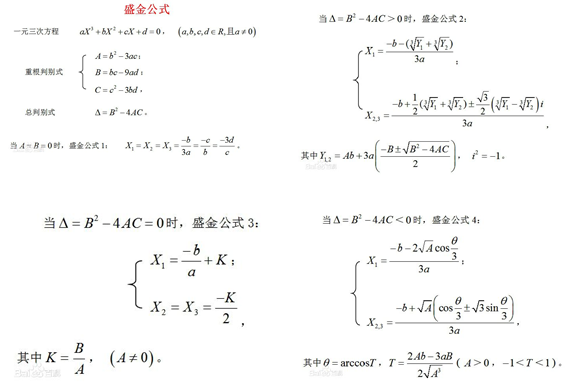①：当A=B=0时，方程有一个三重实根；

②：当Δ=B2－4AC>0时，方程有一个实根和一对共轭虚根；

③：当Δ=B2－4AC=0时，方程有三个实根，其中有一个两重根；

④：当Δ=B2－4AC<0时，方程有三个不相等的实根。

#include<map>
#include<set>
#include<cmath>
#include<queue>
#include<stack>
#include<cstdio>
#include<vector>
#include<cctype>
#include<cstring>
#include<utility>
#include<cstdlib>
#include<iomanip>
#include<iostream>
#include<algorithm>
#define Clear(x) memset(x,0,sizeof(x))
#define fup(i,a,b) for(int i=a;i<b;i++)
#define rfup(i,a,b) for(int i=a;i<=b;i++)
#define fdn(i,a,b) for(int i=a;i>b;i--)
#define rfdn(i,a,b) for(int i=a;i>=b;i--)
typedef long long ll;
using namespace std;
const int maxn = 1e+2;
const int inf = 0x3f3f3f3f;
const double pi=acos(-1.0);
const double eps = 1e-3;
double a,b,c,d;
/**
盛金公式
*/

int main()
{
double x1,x2,x3,temp;
scanf("%lf%lf%lf%lf",&a,&b,&c,&d);
double A=b*b-3*a*c;
double B=b*c-9*a*d;
double C=c*c-3*b*d;
double del=B*B-4*A*C;
//Δ=B2－4AC>0时是虚根
if(A==B&&A==0)
{
x1=x2=x3=-b/(3*a);
}else if(del==0){
x1=-b/a+B/A;
x2=x3=(-B/A)/2;
}else if(del<0){
double T=(2*A*b-3*a*B)/(2*A*sqrt(A));
double _xt=acos(T);
double xt=_xt/3;
x1=(-b-2*sqrt(A)*cos(xt))/(3*a);
x2=(-b+sqrt(A)*(cos(xt)+sqrt(3)*sin(xt)))/(3*a);
x3=(-b+sqrt(A)*(cos(xt)-sqrt(3)*sin(xt)))/(3*a);
}
printf("%.2lf %.2lf %.2lf\n",x1,x2,x3);
return 0;
}


# 牛顿迭代法（求三个根）

首先 f(x)=ax3+bx2+cx+d 求导得到 df/dx=3ax2+2bx+c
求这个导数的零点(就是二次函数求根公式了)得到f(x)的最值点
最值点组成的三个区间一定各有一个f(x)零点，使用牛顿迭代法求得这个零点即可
牛顿迭代法就是不停的用一个点的切线拟合曲线，那个点的导数就是切线斜率

依次类推，可以得到求高次函数零点的一种迭代法：
求n次函数零点，需要极值点来划分区间，也就需要求其导数(n-1次函数)的零点，依次迭代到n=2直接通过公式(当然n=3或4也可以)
最后的复杂度依赖于求零点算法的复杂读



#include <iostream>
#include <cstdio>
#include <cstring>
#include <cmath>
#include <algorithm>
using namespace std;
const double eps=1e-3;
double a,b,c,d;
inline double f(double x){return ((a*x+b)*x+c)*x+d;}
inline double df(double x){return (3*a*x+2*b)*x+c;}
double sol(double l,double r){//printf("sol %lf %lf\n",l,r);
int step=20;double x=(l+r)/2;
while(step--){
x=x-f(x)/df(x);
}
return x;
}
int main(int argc, const char * argv[]) {
scanf("%lf%lf%lf%lf",&a,&b,&c,&d);
double p1=(-sqrt(b*b-3*a*c)-b)/(3*a),
p2=(+sqrt(b*b-3*a*c)-b)/(3*a);
printf("%.2f %.2f %.2f\n",sol(-100,p1),sol(p1,p2),sol(p2,100));
return 0;
}

百度百科

# 韦达定理

由代数基本定理加上数学归纳法可推出其能分解成a(x-x1)(x-x2)(x-x3)的形式（x1,x2,x3∈复数域）

x1x2x3=-(d/a)

x1x2+x2x3+x1x3=c/a

x1+x2+x3=-b/a

这就是三次方程时的韦达定理

展开全文• 用MATLAB实现最小二乘法一元线性拟合，并求出预测直线的斜率与截距。
• 这其中也有别的原因的，高中时候也研究过一元次方程，但是当时绞尽脑汁也没有想出求解的办法，只是找出了三个根之间的关系，于是很不甘心，之后虽然也百度到了解答，但是由于步骤太过复杂冗长导致完全看不进去，...C语言 二分法
• (1)关系:k=tanα 中,k——斜率 α——倾斜角 (2)当斜率大于0时,斜率越大,倾斜角越大.当斜率小于0时,斜率越大,倾斜角越大.当斜率符号不相同时,负的比正的大。一次函数中。k的大小对函数图像有什么影响?k指的是函数...
• matlab 已知二函数系数 怎么画出二函数图像x*x不正确,你是需要计算x的平方对吧,那么需要x.*x或者x.^2,点乘如果是x*x...已知二次方程式函数图像,怎么画出对应的导数图像?1、二函数的导数是一次函数,那么其导数...
• 1.一元n非线性方程 1.1非线性函数 1.2 非线性函数案例 1.3 非线性函数的几何图形 2.扭断迭代法求非线性方程解的基本原理 2.1 概述 2.2确定误差或收敛条件 2.3迭代过程 2.3 二分法的优缺点 3. Pytho...
• 有形如：ax3+bx2+cx+d=0 这样的一元次方程。给出该方程中各项的系数(a，b，c，d 均为实数)，并约定该方程存在三个不同实根(根的范围在-100至100之间)，且根与根之差的绝对值&amp;gt;=1。要求由小到大依次...算法 c++
• 2018-03-05在二函数Y=aX^2 bx c中，a代表什么，b，c都代表什么哪些图像性质? 二函数在图像上有什么概念和性质?y=ax^2 bx c在数学中，二函数(quadratic ... 二函数表达式ax2 bx c的定义是个二多项式，因...
• 一次函数是很多最早学习的函数知识内容之，它的图像是条直线，而学好一次函数，那么首先要掌握好一元一次方程、二元一次方程、二元一次方程组等相关知识内容。从某种意义上来说，直线方程的概念本质上是刻画直线...
• 无论时代再如何变，人类的知识体系永远不会变，除非这个世界本身就不是真实的。...找了许许多多的方案，最终决定尝试种方案，天下来没做啥，真的累了，数据更直观，用相应的公式和数据进行分...
• 有形如：ax3+bx2+cx+d=0 这样的一元次方程。给出该方程中各项的系数(a，b，c，d 均为实数)，并约定该方程存在三个不同实根(根的范围在-100至100之间)，且根与根之差的绝对值&gt;=1。要求由小到大依次在...
• 机器学习1:一元线性回归 原理 一元线性回归 y = b + k????...这个方程对应的图像是条直线，称作回归线。...梯度下降法求一元回归方程斜率和截距 学习率不能太小，也不能太大，可以多尝试一些值 0.1,0.03,0.01,0...机器学习
• 开发过程中用不到一元一次方程吗?非也,iOS开发中经常会遇到根据某个ScrollView动态偏移量的值来实时设置个View的透明度,你敢说你不用一元一次方程你能搞定? 想把个动画效果做好,经常会遇到实时设置的问题,本人...
• 思路：一元次方程有3个不相同的根，说明曲线必然是下面俩种情况中的其中种， 那我们的思路就很清晰了，构造个三函数，f(x) = ax^3 + b * x^2 + cx + d，对这个三函数进行求导，导数为0的所对应的点为极值...二分法 算法
• plot是绘制一元曲线的基本函数，它利用自变量的系列数据和应变量的值进行绘图。 1）举例画条正弦曲线： x = linspace(0,2*pi,100); y = sin(x); plot(x,y); 2）若要画多条曲线只需要将对应坐标依次放入plot...matlab
• 使用一元次方程做实时动画 效果: 原理(图中坐标略有错误,仅供参考-_-!!): YXMath.h + YXMath.m // // YXMath.h // // http://home.cnblogs.com/u/YouXianMing/ // // Copyright (c) 2014年 Y.X. All rights ...
• 最近在写个荧光图像分析软件，需要自己拟合方程一元回归线公式的算法参考了《Java数值方法》，拟合度R^2(绝对系数)是自己写的，欢迎讨论。计算结果和Excel完全一致。总共三个文件：DataPoint.java/*** A data ...
• # 导入sklearn库下的linear_...# 一元线性回归 # 转化自变量数据为矩阵 x = [, , , , ] y = [, , , [17.5], ] # 现在只能用二元矩阵 # print(x) # print(y) # 调用线性回归函数 c...
• 根据多组数据(x,y)模拟得到一次线性方程(斜率和截距)，然后输入新的x来智能预测y值 目录 输出结果 代码设计 输出结果 代码设计 import numpy as np def fitSLR(x,y): n=len(x) ...
• 《统计学(第六版)》贾俊平 第11章 一元线性回归 变量之间的关系可以分为两种类型，即函数关系和相关关系。 函数关系 functional relationship 函数关系是一一对应的确定关系。设有两个变量x和y，变量y随变量x一起...回归分析
• 而数据集最终可以总结出个线性公式，即一元函数 y=h(x)=kx+by=h(x)=kx+by=h(x)=kx+b 。那么我们的目标是让通过对两个参数 kkk 和 bbb 的调整，将 yyy 的实际值和模型输出预测值的误差尽量的小。 用最小二乘法...python 算法
• 个参数为截距，第二个参数为斜率 为了求解上述参数，我们在这里引入代价函数（cost function）,在这里以一元线性回归模型为例： 上述子为真实值与预测值之间的差值的平方（当然也可以取绝对值，但为便于...机器学习 线性回归
• 简述线性回归模型是机器学习里面最基础的种模型，是为了解决回归问题，学习机器学习从线性回归开始最好，网上关于机器...假设函数、损失函数和梯度下降法首先，我们利用sklearn包来生成一元回归数据集impor...
• 目 录 1.σ2的估计 2.回归方程的显著性检验 ...、σ2 的估计 　因为假设检验以及构造与回归模型有关的区间估计都需要σ2的估计量，所以先对σ2作估计。 　通过残差平方和（误差平方和） （1） （用到......